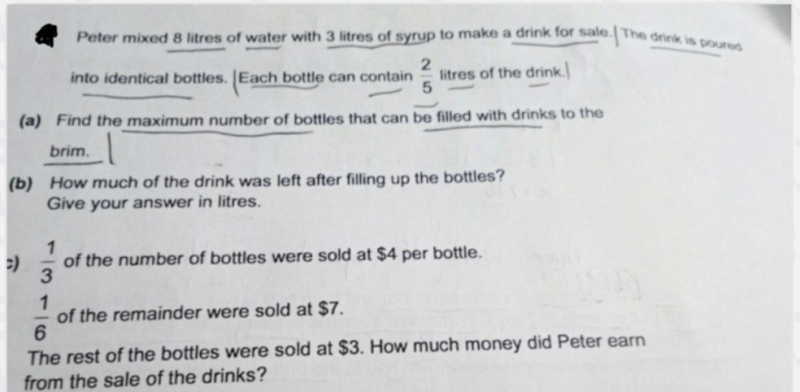# Question(a) Total litres = 8+3 = 11

11 / (2/5) = 27.5

So maximum bottles filled to the brim = 27

(b) Remaining drink left = 11 – [27 x (2/5)] = 11 – 10.8 = 0.2 litres or (1/5) litres

(c) 1/3 of bottles = 1/3 x 27 = 9                     9×4 = 36

1/6 of remaining = 1/6 x 18 = 3              3 x 7 = 21

remaining bottles = 15                             15 x 3  = 45

Hence Peter earned 36 + 21 + 45 = \$102

0 Replies 1 Like